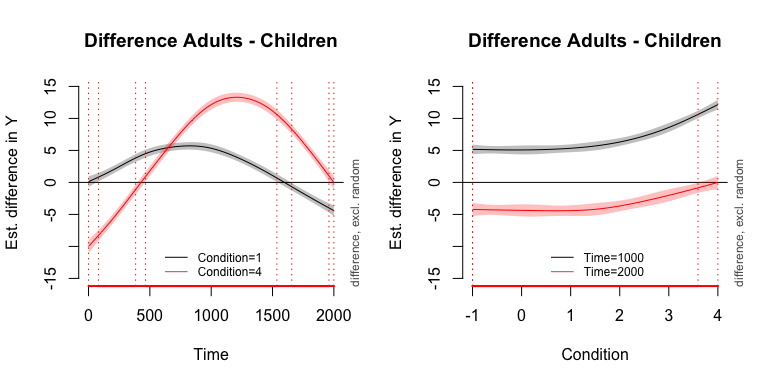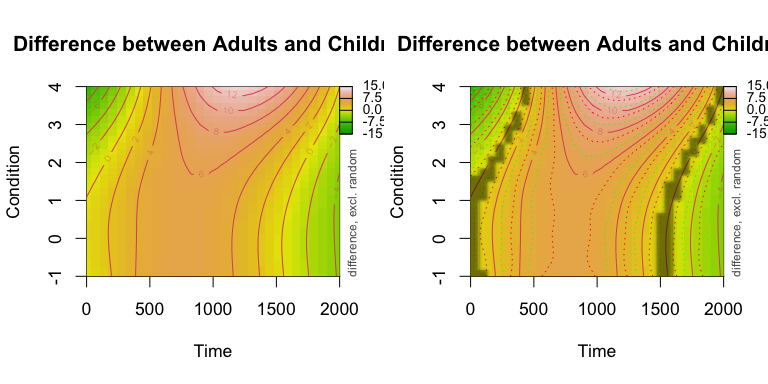# Testing for significance

#### 15 March 2016

Generally, there are three methods to test whether a certain predictor or interaction is significantly contributing to the model’s account of the data:

1. Model comparison procedure

2. Inspection of the summary

3. Visual inspection of the model’s estimates

## Example

Loading the data:

library(itsadug)
library(mgcv)
data(simdat)
# select subset of data to reduce processing time:
select <- 1:18
select <- select[select %% 3 ==0]

## 1. Model comparison

To test whether the three-way interaction between Time, Condition and Group is significant, we can compare the model with a model that does not include this three-way interaction:

m2Rho <- bam(Y ~ Group + s(Time, by=Group) + s(Condition, by=Group, k=5) + ti(Time, Condition) + s(Time, Subject, bs='fs', m=1, k=5) + s(Event, bs='re'), data=simdat, method="fREML", AR.start=simdat$start.event, rho=r1) #### Function compareML The function compareML compares two models on the basis of the minimized smoothing parameter selection score specified in the model, and performes a $$\chi^2$$ test on the difference in scores and the difference in degrees of freedom. # make sure that info messages are printed to the screen: infoMessages('on') compareML(m1Rho, m2Rho) ## m1Rho: Y ~ Group + s(Time, by = Group) + s(Condition, by = Group, k = 5) + ## ti(Time, Condition, by = Group) + s(Time, Subject, bs = "fs", ## m = 1, k = 5) + s(Event, bs = "re") ## ## m2Rho: Y ~ Group + s(Time, by = Group) + s(Condition, by = Group, k = 5) + ## ti(Time, Condition) + s(Time, Subject, bs = "fs", m = 1, ## k = 5) + s(Event, bs = "re") ## ## Chi-square test of fREML scores ## ----- ## Model Score Edf Difference Df p.value Sig. ## 1 m2Rho 43415.70 16 ## 2 m1Rho 43393.79 19 21.901 3.000 1.663e-09 *** ## ## AIC difference: -10.54, model m1Rho has lower AIC. The following conclusions can be derived from the output: • Model m1Rho has a lower fREML score (lower indicates better fit). • But model m1Rho is also more complex: it uses more degrees of freedom (Edf). Note that Edf in the model comparison are different from the edf that are presented in the model summary. The first are reflecting the complexity of the model (number of model terms, complexity of model terms), and the second are reflecting the complexity of the smooth or surface pattern (i.e., number of knots or underlying base functions used). • Model m1Rho is preferred, because the difference in fREML is significant given the difference in degrees of freedom: $$\chi^2$$(3)=21.836, p < .001. #### Some notes on model comparison Model comparison procedure provides an indication for the best fitting model, but can rarely used on it’s own for determining significance. • For testing the difference in fixed effects predictors the method fREML does not provide the most reliable test. Rather use ML. However, ML takes longer to run (that is why it is not included here), and penalizes wigglyness more. • An alternative test is AIC, but when an AR1 model is included, AIC does not provide a reliable test. (Like here!) AIC(m1Rho, m2Rho) ## df AIC ## m1Rho 232.8363 86485.24 ## m2Rho 244.0608 86495.78 ## 2. Inspection of the model summary Beside model comparison the model summary (e.g., summary(m1Rho)) could provide useful information on whether or not a model term is significantly contributing to the model. To include the summary in a R markdown or knitr report use the function gamtabs: gamtabs(m1Rho, type="HTML")  A. parametric coefficients Estimate Std. Error t-value p-value (Intercept) 2.0815 0.0782 26.6229 < 0.0001 GroupAdults 3.1233 0.1106 28.2475 < 0.0001 B. smooth terms edf Ref.df F-value p-value s(Time):GroupChildren 8.1701 8.8251 1883.6351 < 0.0001 s(Time):GroupAdults 8.5790 8.9528 3696.9128 < 0.0001 s(Condition):GroupChildren 3.5242 3.6217 226.3898 < 0.0001 s(Condition):GroupAdults 3.7460 3.8068 481.0384 < 0.0001 ti(Time,Condition):GroupChildren 15.4474 15.9501 1275.5365 < 0.0001 ti(Time,Condition):GroupAdults 15.3403 15.9282 845.9445 < 0.0001 s(Time,Subject) 0.0010 56.0000 0.0000 0.8756 s(Event) 174.3143 248.0000 2.4927 < 0.0001 The summary provides the following information: • There is an overall difference in Y for children and adults (parametric terms) • The F values / p-values of the ‘fixed’ effects smooth terms indicate that all these smooth terms are significantly different from 0, so each line or surface is significantly wiggly. • However, we can NOT conclude that the lines or surfaces are different from each other. This is only possible when we would use difference smooths or tensors, with ordered factors or binomial predictors. See below for an example. • For the random effects the statistics indicates whether or not these terms contribute to the model (s(Event)) or not (s(Time,Subject)). #### Using ordered factors (advanced) It is possible to change the contrasts for grouping predictors in mgcv so that the smooth terms represent differences with the reference level, similar to the treatment coding used in lmer or in the summary of parametric terms in GAMMs. The trick is to first convert the factors to ordered factors so that gam() and bam() won’t use the default contrast coding. Here’s an example: simdat$OFGroup <- as.ordered(simdat$Group) contrasts(simdat$OFGroup) <- "contr.treatment"
contrasts(simdat$OFGroup) ## Adults ## Children 0 ## Adults 1 Note that in the case of using ordered factors we need to include the reference curves or surfaces as well. m1Rho.OF <- bam(Y ~ OFGroup + s(Time) + s(Time, by=OFGroup) + s(Condition, k=5) + s(Condition, by=OFGroup, k=5) + ti(Time, Condition) + ti(Time, Condition, by=OFGroup) + s(Time, Subject, bs='fs', m=1, k=5) + s(Event, bs='re'), data=simdat, method="fREML", AR.start=simdat$start.event, rho=r1)

With the ordered factors suddenly the lines s(Time):OFGroupAdults and similar lines represent the difference between the adults and the reference group, the children. When the smooth term is significant, the difference smooth is is significantly different from zero. So that means that the two groups are different from each other:

gamtabs(m1Rho.OF, type="HTML")
 A. parametric coefficients Estimate Std. Error t-value p-value (Intercept) 2.0813 0.0781 26.6581 < 0.0001 OFGroupAdults 3.1236 0.1104 28.2922 < 0.0001 B. smooth terms edf Ref.df F-value p-value s(Time) 8.2883 8.7974 1891.3882 < 0.0001 s(Time):OFGroupAdults 7.3870 8.3331 409.8352 < 0.0001 s(Condition) 3.6481 3.7167 224.6983 < 0.0001 s(Condition):OFGroupAdults 2.8349 2.9713 34.0186 < 0.0001 ti(Time,Condition) 15.5467 15.9140 1280.8817 < 0.0001 ti(Time,Condition):OFGroupAdults 13.1446 14.9445 129.8732 < 0.0001 s(Time,Subject) 0.0015 56.0000 0.0000 0.7355 s(Event) 174.7125 248.0000 2.4914 < 0.0001

In summary, with continuous predictors or ordered factors we can use the summary startistics to determine the difference of smooth terms.

The function report_stats describes how one could report the smooth terms in the text of an article:

report_stats(m1Rho.OF)

## 3. Visual inspection of the model’s estimates

#### Function plot_diff

The function plot_diff allows to plot the (1 dimensional) estimated difference between two conditions. The argument rm.ranef=TRUE indicates that random effects should be excluded first, and the argument cond can be used to specify values for other predictors.

The plots below visualize the difference between adults and children.

par(mfrow=c(1,2))

# PLOT 1:
plot_diff(m1Rho, view="Time", comp=list(Group=c("Adults", "Children")), cond=list(Condition=1), rm.ranef=TRUE, ylim=c(-15,15))
plot_diff(m1Rho, view="Time", comp=list(Group=c("Adults", "Children")),  cond=list(Condition=4), add=TRUE, col='red')
# add legend:
legend('bottom', legend=c("Condition=1", "Condition=4"), col=c(1,2), lwd=1, cex=.75, bty='n')

# PLOT 2:
plot_diff(m1Rho, view="Condition", comp=list(Group=c("Adults", "Children")), cond=list(Time=1000), rm.ranef=TRUE, ylim=c(-15,15))
plot_diff(m1Rho, view="Condition", comp=list(Group=c("Adults", "Children")),  cond=list(Time=2000), add=TRUE, col='red')
# add legend:
legend('bottom', legend=c("Time=1000", "Time=2000"), col=c(1,2), lwd=1, cex=.75, bty='n')#### Function plot_diff2

The function plot_diff allows to plot the (2 dimensional) estimated difference between two conditions. The argument rm.ranef=TRUE indicates that random effects should be excluded first, and the argument cond can be used to specify values for other predictors.

The plots below visualize the difference between adults and children.

par(mfrow=c(1,2), cex=1.1)
plot_diff2(m1Rho, view=c("Time", "Condition"), comp=list(Group=c("Adults", "Children")), zlim=c(-15,15), se=0, rm.ranef=TRUE)
## Warning in gradientLegend(zlim, n.seg = 3, pos = 0.875, dec = dec, color =
## color, : Increase right margin to fit labels or decrease the number of decimals,
## see help(gradientLegend).
# with CI:
plot_diff2(m1Rho, view=c("Time", "Condition"), comp=list(Group=c("Adults", "Children")), zlim=c(-15,15), se=1.96, rm.ranef=TRUE, show.diff = TRUE)
## Warning in gradientLegend(zlim, n.seg = 3, pos = 0.875, dec = dec, color =
## color, : Increase right margin to fit labels or decrease the number of decimals,
## see help(gradientLegend).